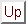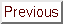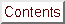Next: Acknowledgments - Remerciements Up: Thesis Constrained, non-linear, derivative-free Previous: Thesis Constrained, non-linear, derivative-free   Contents

# Summary

This thesis is about parallel, direct and constrained optimization of high-computing-load objective functions.

The main result is a new original algorithm: CONDOR ('' COnstrained, Non-linear, Direct, parallel Optimization using trust Region method for high-computing load, noisy functions''). Other original results are described at the beginning of Chapter 1. The function of this algorithm is to find the minimum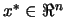of an objective function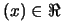using the least number of function evaluations. It is assumed that the dominant computing cost of the optimization process is the time needed to evaluate the objective function(One evaluation can range from 2 minutes to 2 days). The algorithm will try to minimize the number of evaluations of, at the cost of a huge amount of routine work.

CONDOR has been designed with one application in mind: the METHOD project. (METHOD stands for Achievement Of Maximum Efficiency For Process Centrifugal Compressors THrough New Techniques Of Design). The goal of this project is to optimize the shape of the blades inside a Centrifugal Compressor (see illustration of the compressor's blades in Figure 11.1).The objective function is based on a CFD (computation fluid dynamic) code which simulates the flow of the gas inside the compressor. The shape of the blades in the compressor is described by 31 parameters (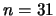). We extract from the numerical simulation the outlet pressure, the outlet velocity, the energy transmit to the gas at stationary conditions. We aggregate all these indices in one general overall number representing the quality of the turbine. We are trying to find the optimal set of 31 parameters for which this quality is maximum. The evaluations of the objective function are very noisy and often take more than one hour to complete (the CFD code needs time to converge'').

CONDOR is a direct optimization tool (i.e., that the derivatives ofare not required). The only information needed about the objective function is a simple method (written in Fortran, C++,...) or a program (a Unix, Windows, Solaris,... executable) which can evaluate the objective functionat a given point. The algorithm has been specially developed to be very robust against noise inside the evaluation of the objective function. This situation is very general, the algorithm can thus be applied on a vast number of situations.

CONDOR is able to use several CPU's in a cluster of computers. Different computer architectures can be mixed together and used simultaneously to deliver a huge computing power. The optimizer will make simultaneous evaluations of the objective functionon the available CPU's to speed up the optimization process.

The experimental results are very encouraging and validate the quality of the approach: CONDOR outperforms many commercial, high-end optimizer and it might be the fastest optimizer in its category (fastest in terms of number of function evaluations). When several CPU's are used, the performances of CONDOR are unmatched.
The experimental results open wide possibilities in the field of noisy and high-computing-load objective functions optimization (from two minutes to several days) like, for instance, industrial shape optimization based on CFD (computation fluid dynamic) codes (see [CAVDB01,PVdB98,Pol00,PMM$^+$03]) or PDE (partial differential equations) solvers.

Finally, we propose a new, original, easily comprehensible, free and fully stand-alone implementation in C/C++ of the optimization algorithm: the CONDOR optimizer. There is no call to fortran, external, unavailable, expensive, copyrighted libraries. You can compile the code under Unix, Windows, Solaris,etc. The only library needed is the standard TCP/IP network transmission library based on sockets (only in the case of the parallel version of the code).

The algorithms used inside CONDOR are part of the Gradient-Based optimization family. The algorithms implemented are Dennis-Moré Trust Region steps calculation (It's a restricted Newton's Step), Sequential Quadratic Programming (SQP), Quadratic Programming(QP), Second Order Corrections steps (SOC), Constrained Step length computation usingmerit function and Wolf condition, Active Set method for active constraints identification, BFGS update, Multivariate Lagrange Polynomial Interpolation, Cholesky factorization, QR factorization and more!

Many ideas implemented inside CONDOR are from Powell's UOBYQA (Unconstrained Optimization BY quadratical approximation) [Pow00] for unconstrained, direct optimization. The main contribution of Powell is Equation 6.6 which allows to construct a full quadratical model of the objective function in very few function evaluations (at a low price). This equation is very successful in that and having a full quadratical model allows us to reach unmatched convergence speed.
A full comprehension of all the aspects of the all the algorithms was, for me, one of the most important point. So I started from scratch and recoded everything. This allowed me to have a fully stand-alone implementation. Full comprehension and full re-implementation is needed to be able to extend the ideas to the parallel and constrained cases.Next: Acknowledgments - Remerciements Up: Thesis Constrained, non-linear, derivative-free Previous: Thesis Constrained, non-linear, derivative-free   Contents
Frank Vanden Berghen 2004-04-19